Ch 1. Particle General Motion Multimedia Engineering Dynamics Position,Vel & Accel. Accel. varyw/ Time Accel. Constant Rect. Coordinates Norm/Tang. Coordinates Polar Coordinates RelativeMotion
 Chapter - Particle - 1. General Motion 2. Force & Accel. 3. Energy 4. Momentum - Rigid Body - 5. General Motion 6. Force & Accel. 7. Energy 8. Momentum 9. 3-D Motion 10. Vibrations Appendix Basic Math Units Basic Equations Sections Search eBooks Dynamics Fluids Math Mechanics Statics Thermodynamics Author(s): Kurt Gramoll ©Kurt GramollDYNAMICS - THEORY In the Position, Velocity, Acceleration section, velocity and acceleration of a particle was determined when the position is given as a function of time. Many times, however, the motion of an object is specified by a given acceleration as a function of time. Then the equation needs to be integrated using the initial or boundary conditions. Indefinite Integral If the acceleration is known as a function of time      a(t) = dv/dt      ==>    dv = a(t) dt the velocity can be determined by integrating, giving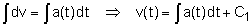a(t) = dv/dt      ==>    dv = a(t) dt The velocity can then be integrated to determine the position as a function of time.Here, the arbitrary constants of integration C1 and C2 appear because there are infinite motions that correspond to a given acceleration. To uniquely determine the values for C1 and C2, the initial conditions or limits of integration must be specified. Definite Integrals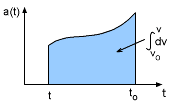Velocity Integration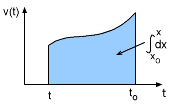position Integration To uniquely determine the motion, the indefinite integrals need to be replaced with definite integrals, setting the lower limits of integration equal to the initial conditions. Integrate the acceleration gives the velocity as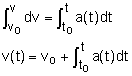From this equation, and the figure at the left, it can be seen that the area defined by the graph of the acceleration is equal to the change in velocity from to to t. Similarly, integrating the velocity will give the position as,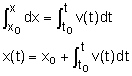From this equation, and the figure at the left, it can be seen that the area defined by the graph of the velocity is equal to the change in position from to to t.

Practice Homework and Test problems now available in the 'Eng Dynamics' mobile app
Includes over 400 problems with complete detailed solutions.
Available now at the Google Play Store and Apple App Store.